Get inspired by the success stories of our students in IIT JAM MS, ISI  MStat, CMI MSc DS.  Learn More

# Eigen Values | ISI MStat 2019 PSB Problem 2This post based on eigen values of matrices and using very basic inequalities gives a detailed solution to ISI M.Stat 2019 PSB Problem 2.

## Problem

Let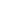and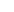be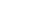matrices. Suppose thathas eigenvalues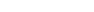andhas eigenvalues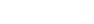where each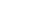(a) Prove that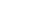has at least one eigenvalue greater than 2.
(b) Prove that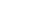has at least one eigenvalue greater than 0.
(c) Give an example ofandso that 1 is not an eigenvalue of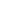.

### Prerequisites

• Trace of a Matrix
• AM - GM Inequality
• Pigeon Hole Principle: Let the average of a set of positive numbers be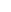. Use the pigeonhole principle to show that there exists at least one number less than or equal to.
• Algebra of Diagonal Matrices
• Eigenvalues of a Diagonal Matrix are the diagonal elements.

## Solution

(a)

Consider the trace of.

Mean of the eigen values of+=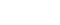=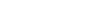=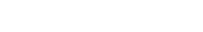=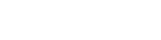. [ Hint: AM - GM Inequality ].

Now, use Pegion Hole Principle as mentioned in the prerequisites.

(b)

Mean of the eigen values of-====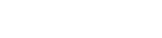. [ Hint: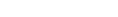.]

Now, use Pegion Hole Principle as mentioned in the prerequisites.

(c)

Let's take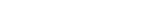and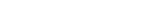. Now observe thatandsatisfy the given conditions.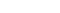. But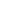has an eigenvalue 1.

So, what to do? We want none of the diagonal values ofto be not 1.

Take,and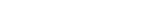. ow observe thatandsatisfy the given conditions.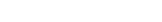, which has no eigen value 1.

Stay tuned!

This post based on eigen values of matrices and using very basic inequalities gives a detailed solution to ISI M.Stat 2019 PSB Problem 2.

## Problem

Letandbematrices. Suppose thathas eigenvaluesandhas eigenvalueswhere each(a) Prove thathas at least one eigenvalue greater than 2.
(b) Prove thathas at least one eigenvalue greater than 0.
(c) Give an example ofandso that 1 is not an eigenvalue of.

### Prerequisites

• Trace of a Matrix
• AM - GM Inequality
• Pigeon Hole Principle: Let the average of a set of positive numbers be. Use the pigeonhole principle to show that there exists at least one number less than or equal to.
• Algebra of Diagonal Matrices
• Eigenvalues of a Diagonal Matrix are the diagonal elements.

## Solution

(a)

Consider the trace of.

Mean of the eigen values of+====. [ Hint: AM - GM Inequality ].

Now, use Pegion Hole Principle as mentioned in the prerequisites.

(b)

Mean of the eigen values of-====. [ Hint:.]

Now, use Pegion Hole Principle as mentioned in the prerequisites.

(c)

Let's takeand. Now observe thatandsatisfy the given conditions.. Buthas an eigenvalue 1.

So, what to do? We want none of the diagonal values ofto be not 1.

Take,and. ow observe thatandsatisfy the given conditions., which has no eigen value 1.

Stay tuned!

This site uses Akismet to reduce spam. Learn how your comment data is processed.

### One comment on “Eigen Values | ISI MStat 2019 PSB Problem 2”

1.Akshay says:

We have first prove the eigenvalues of A+B and A-B are real. Also, there are examples online contradicting the first to statements.

### Knowledge Partner